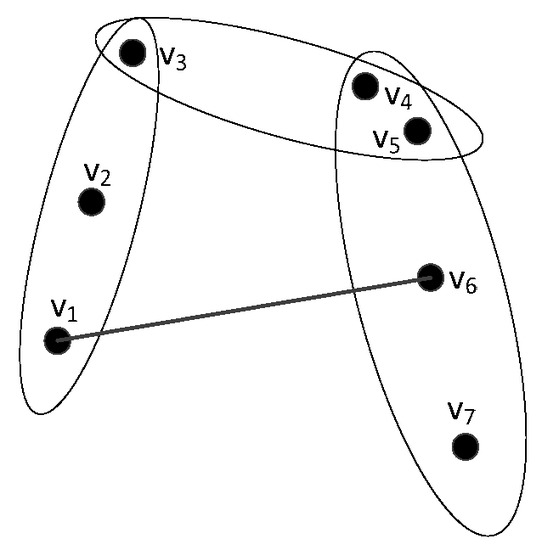Next Article in Journal
The Modified Beta Gompertz Distribution: Theory and Applications
Next Article in Special Issue
The Aα-Spectral Radii of Graphs with Given Connectivity
Previous Article in Journal
On Generalized Closed Sets and Generalized Pre-Closed Sets in Neutrosophic Topological Spaces
Previous Article in Special Issue
Kempe-Locking Configurations

## Volume 7, Issue 1

Article MenuMetrics 0

## Export Article

Open AccessArticle

# The Bounds of the Edge Number in Generalized Hypertrees

1,2,3,*, 2,3,4, 1,2,3 and
1
School of Computer, Qinghai Normal University, Xining 810008, China
2
Key Laboratory of Tibetan Information Processing and Machine Translation in QH, Xining 810008, China
3
Key Laboratory of the Education Ministry for Tibetan Information Processing, Xining 810008, China
4
School of Computer Science, Shannxi Normal university, Xi’an 710062, China
5
School of mathematics and statistics, Qinghai Normal University, Xining 810008, China
*
Author to whom correspondence should be addressed.
Mathematics 2019, 7(1), 2; https://doi.org/10.3390/math7010002
Received: 11 November 2018 / Revised: 14 December 2018 / Accepted: 15 December 2018 / Published: 20 December 2018
(This article belongs to the Special Issue Graph-Theoretic Problems and Their New Applications)
|
PDF [2405 KB, uploaded 20 December 2018]
|

# Abstract

A hypergraph $H = ( V , ε )$ is a pair consisting of a vertex set $V$ , and a set $ε$ of subsets (the hyperedges of $H$ ) of $V$ . A hypergraph $H$ is $r$ -uniform if all the hyperedges of $H$ have the same cardinality $r$ . Let $H$ be an $r$ -uniform hypergraph, we generalize the concept of trees for $r$ -uniform hypergraphs. We say that an $r$ -uniform hypergraph $H$ is a generalized hypertree ( $G H T$ ) if $H$ is disconnected after removing any hyperedge $E$ , and the number of components of $G H T − E$ is a fixed value . We focus on the case that $G H T − E$ has exactly two components. An edge-minimal $G H T$ is a $G H T$ whose edge set is minimal with respect to inclusion. After considering these definitions, we show that an $r$ -uniform $G H T$ on $n$ vertices has at least $2 n / ( r + 1 )$ edges and it has at most $n − r + 1$ edges if , and the lower and upper bounds on the edge number are sharp. We then discuss the case that $G H T − E$ has exactly components. View Full-Text
Keywords:
FiguresFigure 1

This is an open access article distributed under the Creative Commons Attribution License which permits unrestricted use, distribution, and reproduction in any medium, provided the original work is properly cited (CC BY 4.0).# Share & Cite This Article

MDPI and ACS Style

Zhang, K.; Zhao, H.; Ye, Z.; Zhu, Y.; Wei, L. The Bounds of the Edge Number in Generalized Hypertrees. Mathematics 2019, 7, 2.

Note that from the first issue of 2016, MDPI journals use article numbers instead of page numbers. See further details here.

1

# Comments

[Return to top]
Mathematics EISSN 2227-7390 Published by MDPI AG, Basel, Switzerland RSS E-Mail Table of Contents Alert
Back to Top